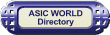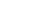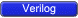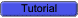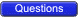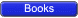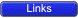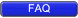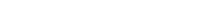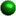Always Blocks As the name suggests, an always block executes always, unlike initial blocks which execute only once (at the beginning of simulation). A second difference is that an always block should have a sensitive list or a delay associated with it.The sensitive list is the one which tells the always block when to execute the block of code, as shown in the figure below. The @ symbol after reserved word ' always', indicates that the block will be triggered "at" the condition in parenthesis after symbol @.One important note about always block: it can not drive wire data type, but can drive reg and integer data types.``` 1 always @ (a or b or sel) 2 begin 3 y = 0; 4 if (sel == 0) begin 5 y = a; 6 end else begin 7 y = b; 8 end 9 end ``` You could download file one_day9.v hereThe above example is a 2:1 mux, with input a and b; sel is the select input and y is the mux output. In any combinational logic, output changes whenever input changes. This theory when applied to always blocks means that the code inside always blocks needs to be executed whenever the input variables (or output controlling variables) change. These variables are the ones included in the sensitive list, namely a, b and sel.There are two types of sensitive list: level sensitive (for combinational circuits) and edge sensitive (for flip-flops). The code below is the same 2:1 Mux but the output y is now a flip-flop output.``` 1 always @ (posedge clk ) 2 if (reset == 0) begin 3 y <= 0; 4 end else if (sel == 0) begin 5 y <= a; 6 end else begin 7 y <= b; 8 end ``` You could download file one_day10.v hereWe normally have to reset flip-flops, thus every time the clock makes the transition from 0 to 1 (posedge), we check if reset is asserted (synchronous reset), then we go on with normal logic. If we look closely we see that in the case of combinational logic we had "=" for assignment, and for the sequential block we had the "<=" operator. Well, "=" is blocking assignment and "<=" is nonblocking assignment. "=" executes code sequentially inside a begin / end, whereas nonblocking "<=" executes in parallel.We can have an always block without sensitive list, in this case we need to have a delay as shown in the code below.``` 1 always begin 2 #5 clk = ~clk; 3 end ``` You could download file one_day11.v here#5 in front of the statement delays its execution by 5 time units.Assign StatementAn assign statement is used for modeling only combinational logic and it is executed continuously. So the assign statement is called 'continuous assignment statement' as there is no sensitive list.``` 1 assign out = (enable) ? data : 1'bz; ``` You could download file one_day12.v hereThe above example is a tri-state buffer. When enable is 1, data is driven to out, else out is pulled to high-impedance. We can have nested conditional operators to construct mux, decoders and encoders.``` 1 assign out = data; ``` You could download file one_day13.v hereThis example is a simple buffer.Task and Function When repeating the same old things again and again, Verilog, like any other programming language, provides means to address repeated used code, these are called Tasks and Functions. I wish I had something similar for webpages, just call it to print this programming language stuff again and again.Code below is used for calculating even parity.``` 1 function parity; 2 input [31:0] data; 3 integer i; 4 begin 5 parity = 0; 6 for (i= 0; i < 32; i = i + 1) begin 7 parity = parity ^ data[i]; 8 end 9 end 10 endfunction ``` You could download file one_day14.v hereFunctions and tasks have the same syntax; one difference is that tasks can have delays, whereas functions can not have any delay. This means that function can be used for modeling combinational logic.A second difference is that functions can return a value, whereas tasks can not.